# 3 4 Linear Programming Objective Solve linear programming

• Slides: 16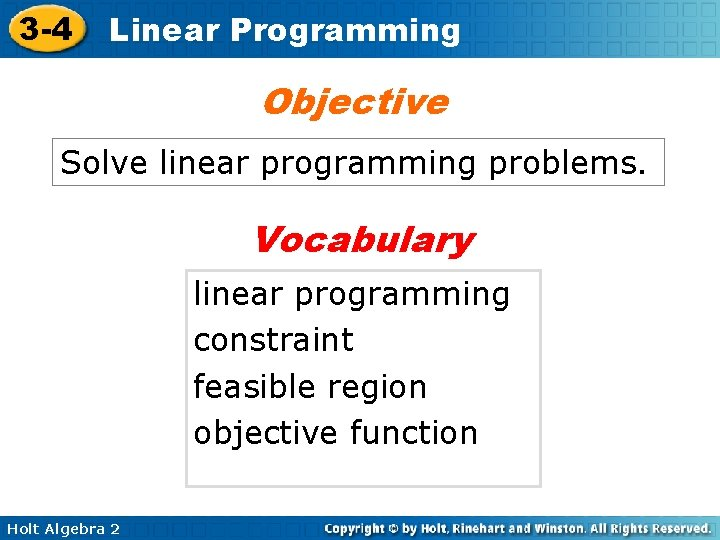3 -4 Linear Programming Objective Solve linear programming problems. Vocabulary linear programming constraint feasible region objective function Holt Algebra 2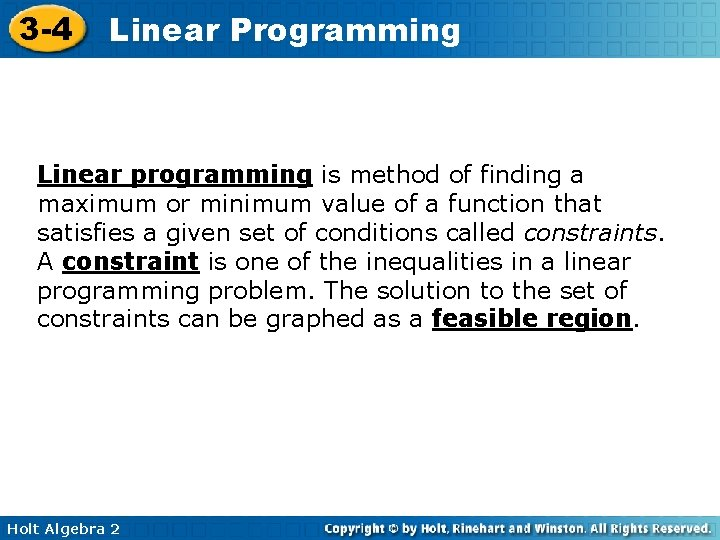3 -4 Linear Programming Linear programming is method of finding a maximum or minimum value of a function that satisfies a given set of conditions called constraints. A constraint is one of the inequalities in a linear programming problem. The solution to the set of constraints can be graphed as a feasible region. Holt Algebra 2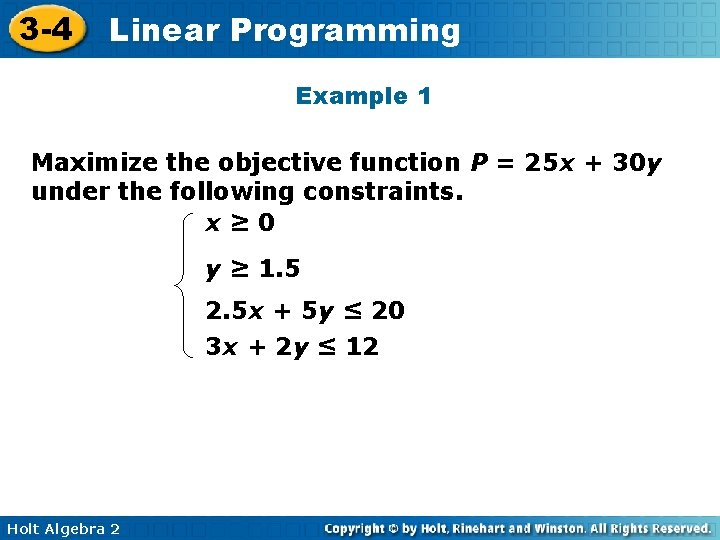3 -4 Linear Programming Example 1 Maximize the objective function P = 25 x + 30 y under the following constraints. x≥ 0 y ≥ 1. 5 2. 5 x + 5 y ≤ 20 3 x + 2 y ≤ 12 Holt Algebra 23 -4 Linear Programming Example 1 Continued Step 1 Write the objective function: P= 25 x + 30 y Step 2 Use the constraints to graph. x≥ 0 y ≥ 1. 5 2. 5 x + 5 y ≤ 20 3 x + 2 y ≤ 12 Holt Algebra 23 -4 Linear Programming Example 1 Continued Step 3 Evaluate the objective function at the vertices of the feasible region. (x, y) (0, 4) (0, 1. 5) 25 x + 30 y 25(0) + 30(4) 25(0) + 30(1. 5) P(\$) 120 45 (2, 3) 25(2) + 30(3) 140 (3, 1. 5) 25(3) + 30(1. 5) 120 P = 140 Holt Algebra 2 The maximum value occurs at the vertex (2, 3).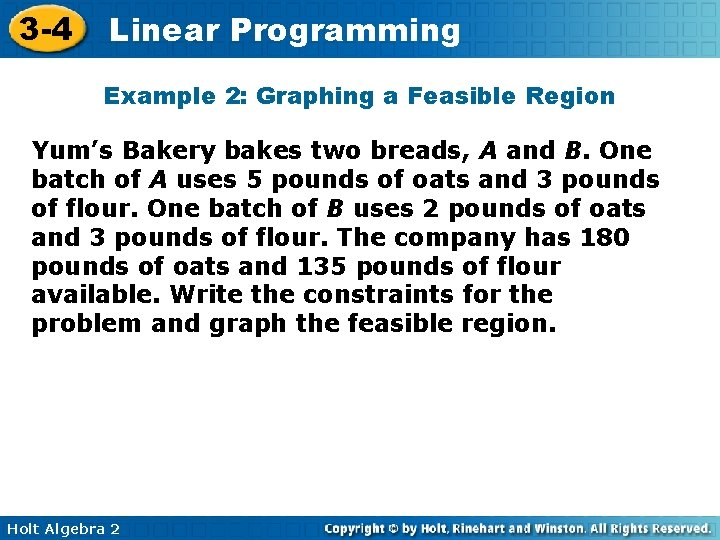3 -4 Linear Programming Example 2: Graphing a Feasible Region Yum’s Bakery bakes two breads, A and B. One batch of A uses 5 pounds of oats and 3 pounds of flour. One batch of B uses 2 pounds of oats and 3 pounds of flour. The company has 180 pounds of oats and 135 pounds of flour available. Write the constraints for the problem and graph the feasible region. Holt Algebra 2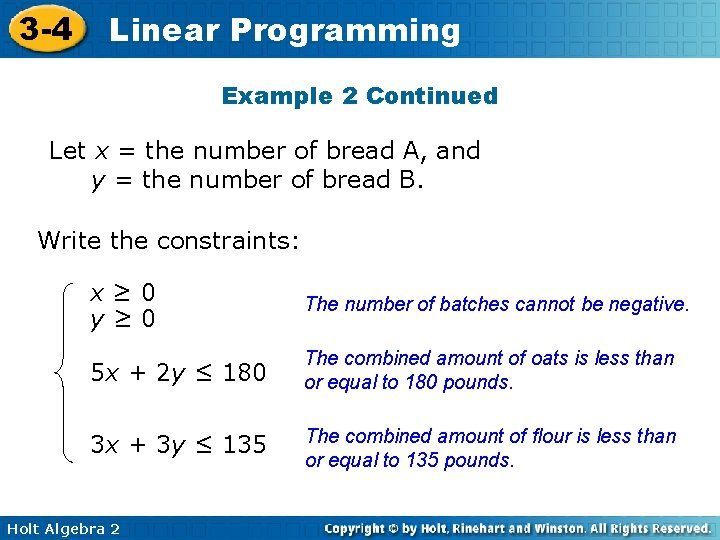3 -4 Linear Programming Example 2 Continued Let x = the number of bread A, and y = the number of bread B. Write the constraints: x≥ 0 y≥ 0 The number of batches cannot be negative. 5 x + 2 y ≤ 180 The combined amount of oats is less than or equal to 180 pounds. 3 x + 3 y ≤ 135 The combined amount of flour is less than or equal to 135 pounds. Holt Algebra 23 -4 Linear Programming Graph the feasible region. The feasible region is a quadrilateral with vertices at (0, 0), (36, 0), (30, 15), and (0, 45). Holt Algebra 2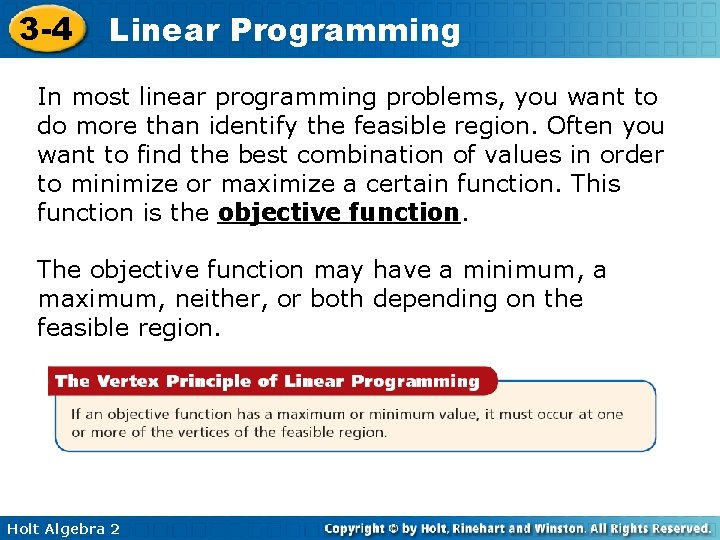3 -4 Linear Programming In most linear programming problems, you want to do more than identify the feasible region. Often you want to find the best combination of values in order to minimize or maximize a certain function. This function is the objective function. The objective function may have a minimum, a maximum, neither, or both depending on the feasible region. Holt Algebra 23 -4 Linear Programming Example 3: Solving Linear Programming Problems Yum’s Bakery wants to maximize its profits from bread sales. One batch of A yields a profit of \$40. One batch of B yields a profit of \$30. Use the profit information and the data from Example 1 to find how many batches of each bread the bakery should bake. Holt Algebra 23 -4 Linear Programming Example 3 continued Step 1 Let P = the profit from the bread. Write the objective function: P = 40 x + 30 y Step 2 Recall the constraints and the graph from Example 1. x≥ 0 y≥ 0 5 x + 2 y ≤ 180 3 x + 3 y ≤ 135 Holt Algebra 23 -4 Linear Programming Example 3 continued Step 3 Evaluate the objective function at the vertices of the feasible region. (x, y) (0, 0) (0, 45) 40 x + 30 y 40(0) + 30(0) 40(0) + 30(45) P(\$) 0 1350 (30, 15) 40(30) + 30(15) 1650 (36, 0) 40(36) + 30(0) 1440 The maximum value occurs at the vertex (30, 15). Yum’s Bakery should make 30 batches of bread A and 15 batches of bread B to maximize the amount of profit. Holt Algebra 2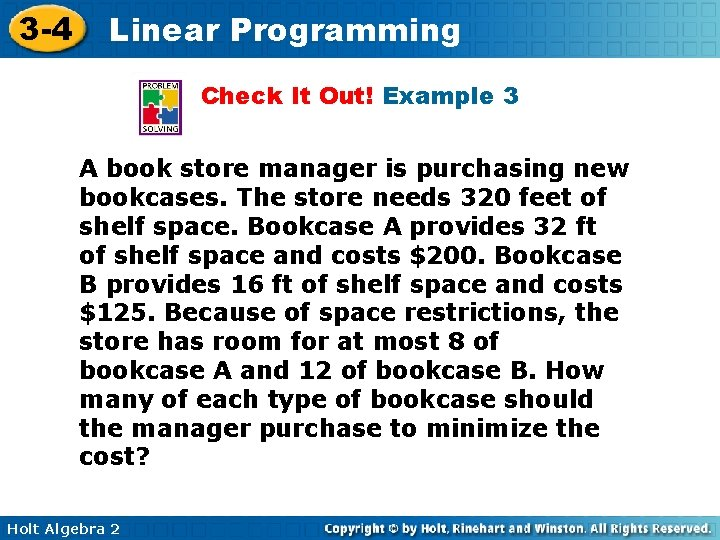3 -4 Linear Programming Check It Out! Example 3 A book store manager is purchasing new bookcases. The store needs 320 feet of shelf space. Bookcase A provides 32 ft of shelf space and costs \$200. Bookcase B provides 16 ft of shelf space and costs \$125. Because of space restrictions, the store has room for at most 8 of bookcase A and 12 of bookcase B. How many of each type of bookcase should the manager purchase to minimize the cost? Holt Algebra 2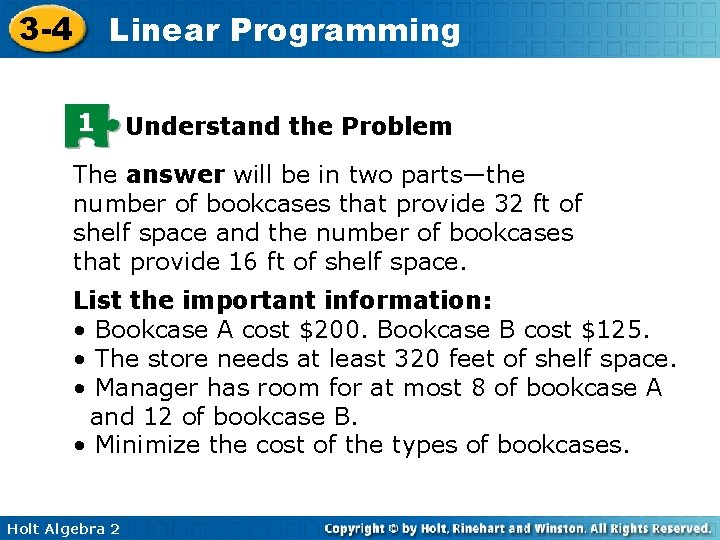3 -4 Linear Programming 1 Understand the Problem The answer will be in two parts—the number of bookcases that provide 32 ft of shelf space and the number of bookcases that provide 16 ft of shelf space. List the important information: • Bookcase A cost \$200. Bookcase B cost \$125. • The store needs at least 320 feet of shelf space. • Manager has room for at most 8 of bookcase A and 12 of bookcase B. • Minimize the cost of the types of bookcases. Holt Algebra 2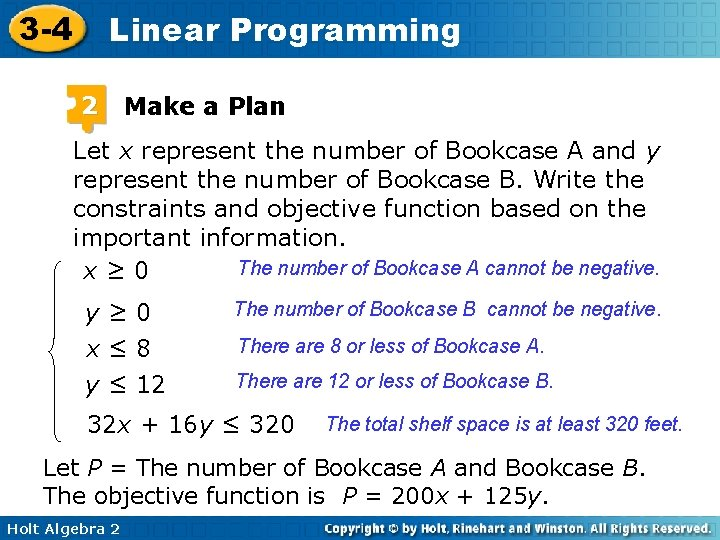3 -4 Linear Programming 2 Make a Plan Let x represent the number of Bookcase A and y represent the number of Bookcase B. Write the constraints and objective function based on the important information. The number of Bookcase A cannot be negative. x≥ 0 y≥ 0 x≤ 8 y ≤ 12 The number of Bookcase B cannot be negative. There are 8 or less of Bookcase A. There are 12 or less of Bookcase B. 32 x + 16 y ≤ 320 The total shelf space is at least 320 feet. Let P = The number of Bookcase A and Bookcase B. The objective function is P = 200 x + 125 y. Holt Algebra 2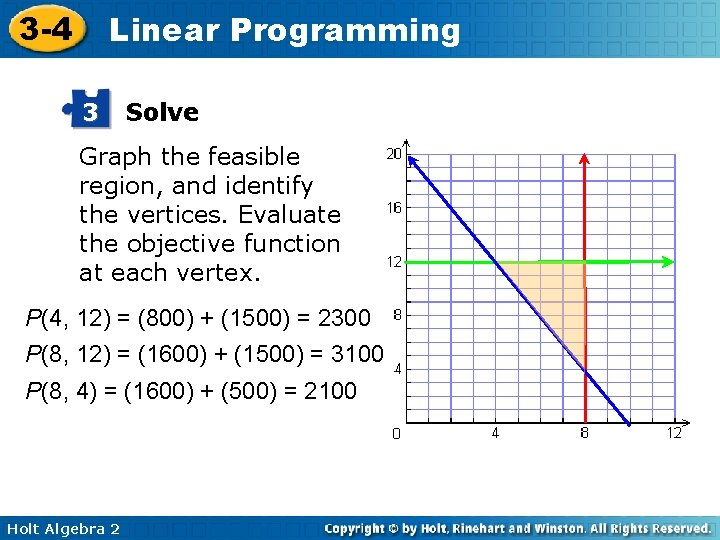3 -4 Linear Programming 3 Solve Graph the feasible region, and identify the vertices. Evaluate the objective function at each vertex. P(4, 12) = (800) + (1500) = 2300 P(8, 12) = (1600) + (1500) = 3100 P(8, 4) = (1600) + (500) = 2100 Holt Algebra 2